# CHAPTER 6 Very Brief introduction to Quantum mechanics

• Slides: 66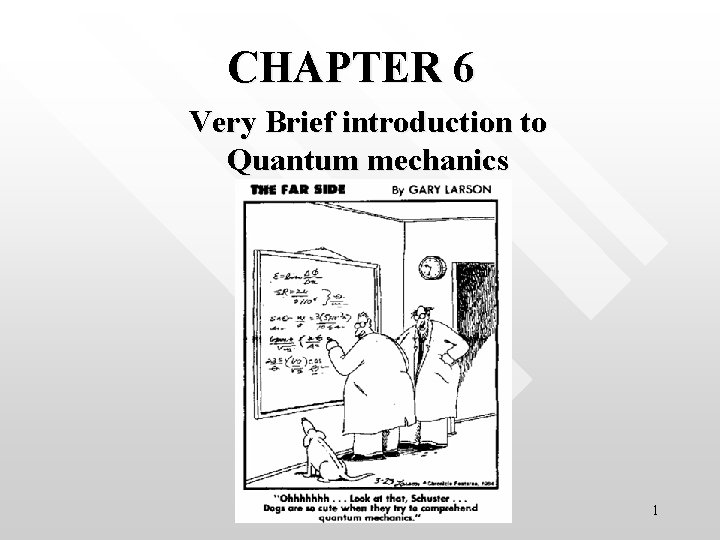CHAPTER 6 Very Brief introduction to Quantum mechanics 1Probabilistic interpretation of matter wave l A=1 unit area A beam of light if pictured as monochromatic wave (l, n) Intensity of the light beam is A beam of light pictured in terms of photons A = 1 unit area E=hn Intensity of the light beam is I = Nhn N = average number of photons per unit time crossing unit area perpendicular to the direction of propagation Intensity = energy crossing one unit area per unit time. I is in unit of joule per m 2 per second 2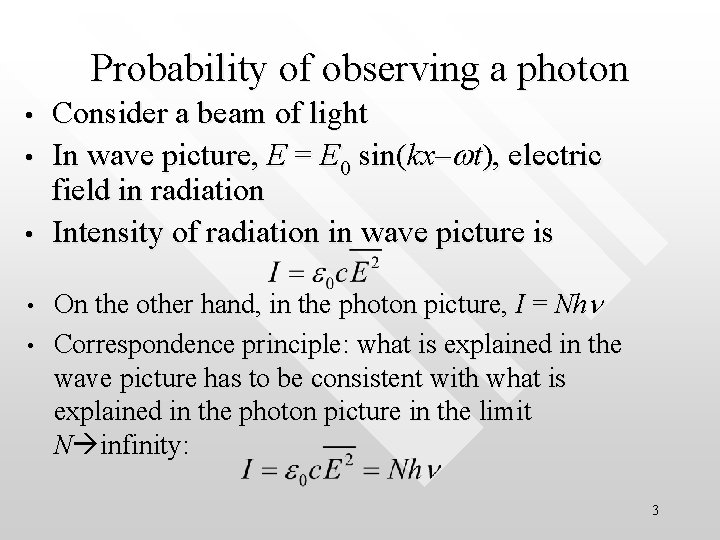Probability of observing a photon • • • Consider a beam of light In wave picture, E = E 0 sin(kx–wt), electric field in radiation Intensity of radiation in wave picture is On the other hand, in the photon picture, I = Nhn Correspondence principle: what is explained in the wave picture has to be consistent with what is explained in the photon picture in the limit N infinity: 3Statistical interpretation of radiation • • The probability of observing a photon at a point in unit time is proportional to N However, since the probability of observing a photon must also This means that the probability of observing a photon at any point in space is proportional to the square of the averaged electric field strength at that point Prob (x) Square of the mean of the square of the wave field amplitude 4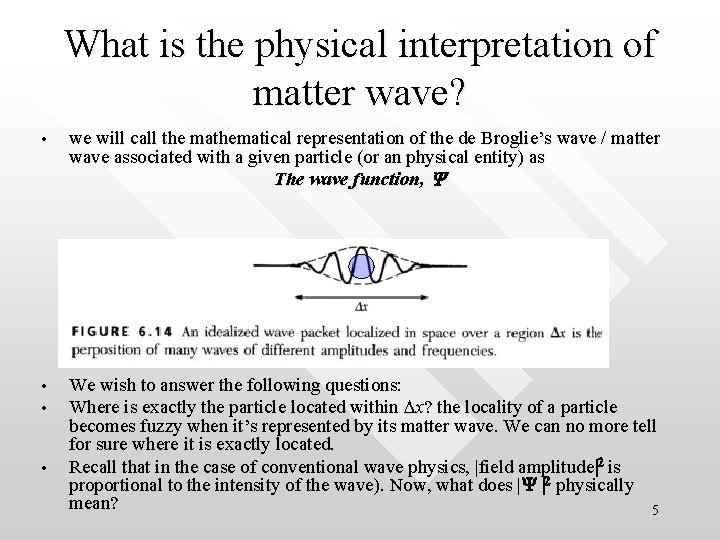What is the physical interpretation of matter wave? • we will call the mathematical representation of the de Broglie’s wave / matter wave associated with a given particle (or an physical entity) as The wave function, Y • We wish to answer the following questions: Where is exactly the particle located within Dx? the locality of a particle becomes fuzzy when it’s represented by its matter wave. We can no more tell for sure where it is exactly located. Recall that in the case of conventional wave physics, |field amplitude|2 is proportional to the intensity of the wave). Now, what does |Y |2 physically mean? 5 • •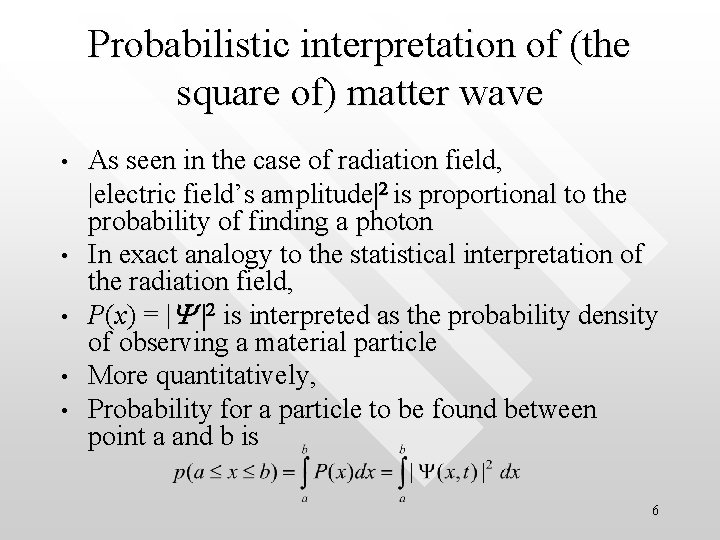Probabilistic interpretation of (the square of) matter wave • • • As seen in the case of radiation field, |electric field’s amplitude|2 is proportional to the probability of finding a photon In exact analogy to the statistical interpretation of the radiation field, P(x) = |Y |2 is interpreted as the probability density of observing a material particle More quantitatively, Probability for a particle to be found between point a and b is 6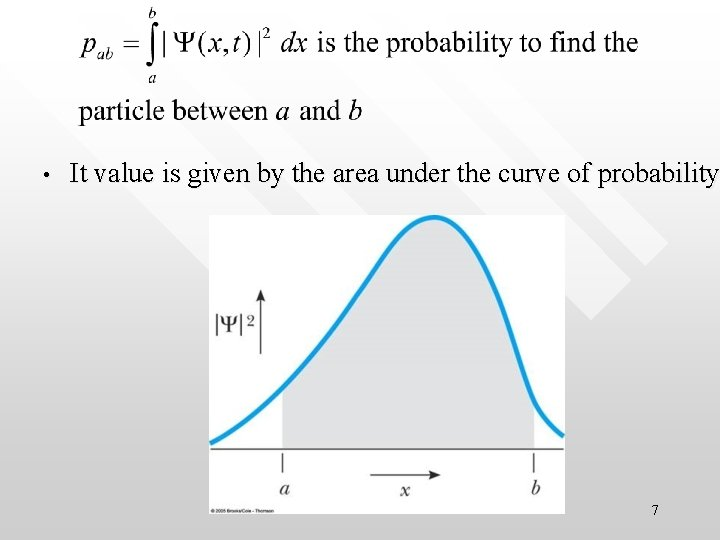• It value is given by the area under the curve of probability 7Expectation value • Any physical observable in quantum mechanics, O (which is a function of position, x), when measured repeatedly, will yield an expectation value of given by • Example, O can be the potential energy, position, etc. (Note: the above statement is not applicable to energy and linear momentum because they cannot be express explicitly as a function of x due to uncertainty principle)… • 8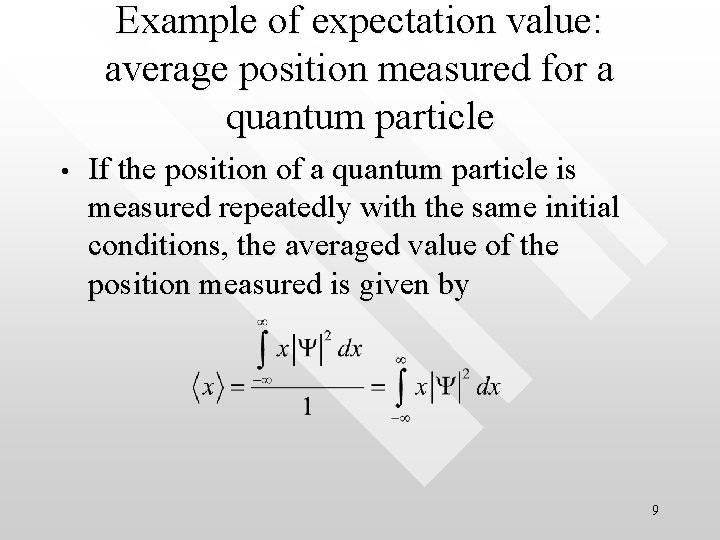Example of expectation value: average position measured for a quantum particle • If the position of a quantum particle is measured repeatedly with the same initial conditions, the averaged value of the position measured is given by 9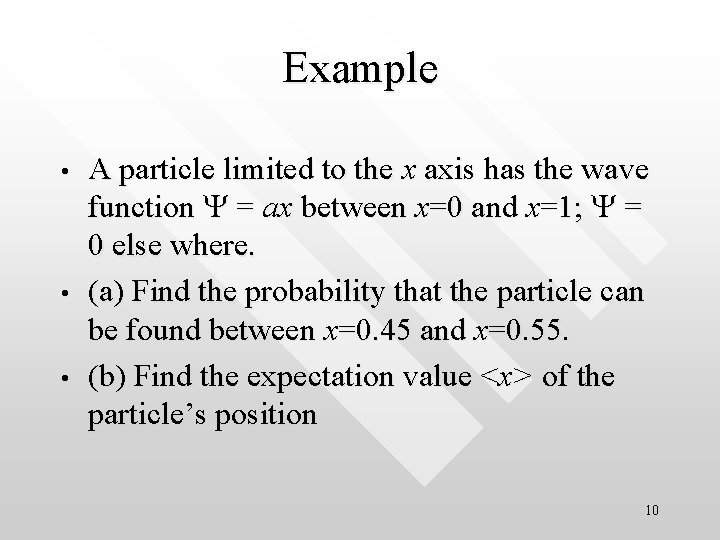Example • • • A particle limited to the x axis has the wave function Y = ax between x=0 and x=1; Y = 0 else where. (a) Find the probability that the particle can be found between x=0. 45 and x=0. 55. (b) Find the expectation value <x> of the particle’s position 10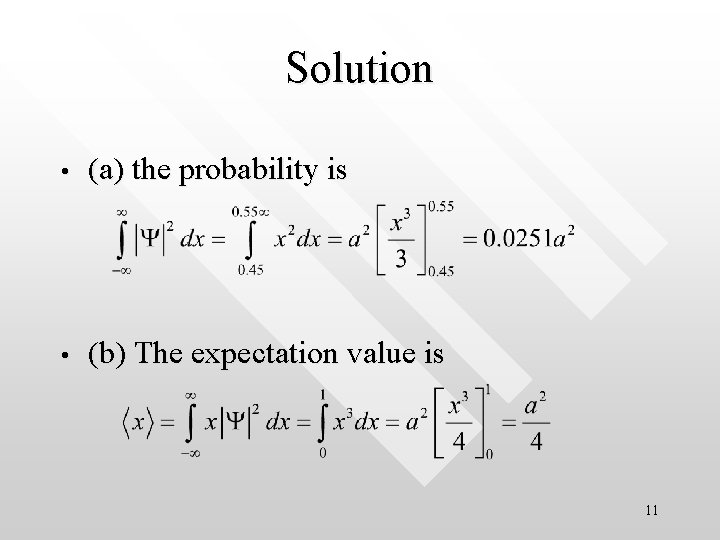Solution • (a) the probability is • (b) The expectation value is 11Max Born and probabilistic interpretation • • Hence, a particle’s wave function gives rise to a probabilistic interpretation of the position of a particle Max Born in 1926 German-British physicist who worked on the mathematical basis for quantum mechanics. Born's most important contribution was his suggestion that the absolute square of the wavefunction in the Schrödinger equation was a measure of the probability of finding the particle at a given location. Born shared the 1954 Nobel Prize in physics with Bethe 12PYQ 2. 7, Final Exam 2003/04 • A large value of the probability density of an atomic electron at a certain place and time signifies that the electron A. is likely to be found there B. is certain to be found there C. has a great deal of energy there D. has a great deal of charge E. is unlikely to be found there • ANS: A, Modern physical technique, Beiser, MCP 25, pg. 802 • • • 13Particle in an infinite well (sometimes called particle in a box) • • • Imagine that we put particle (e. g. an electron) into an “infinite well” In other words, the particle is confined within 0 < x < L In Newtonian view the particle is traveling along a straight line boun 14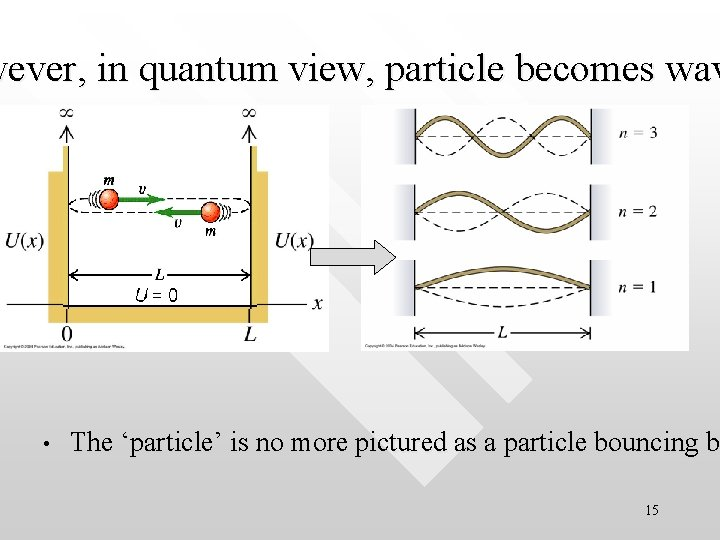wever, in quantum view, particle becomes wav • The ‘particle’ is no more pictured as a particle bouncing be b 15Particle forms standing wave within the infinite well • How would the wave function of the particle behave inside the well? • They form standing waves which are confined within 0≤x≤L 16Standing wave in general • • Shown below are standing waves which ends are fixed at x For standing wave, the speed is constant, (v = l f = constan 17Mathematical description of standing waves • • • In general, the equation that describes a standing wave (with a constant width L) is simply: L = nln/2 n = 1, 2, 3, … (positive, discrete integer) n characterises the “mode” of the standing wave n = 1 mode is called the ‘fundamental’ or the first harmonic n = 2 is called the second harmonics, etc. ln are the wavelengths associated with the n-th mode standing waves The lengths of ln is “quantised” as it can take only discrete values according to ln= 2 L/n 18Energy of the particle in the box • Recall that • For such a free particle that forms standing waves in the box, it has no potential energy • It has all of its mechanical energy in the form of kinetic energy only Hence, for the region 0 < x < L , we write the total energy of the particle as • E = K + V = p 2/2 m + 0 = p 2/2 m 19Energies of the particle are quantised • Due to the quantisation of the standing wave (which comes in the form of ln = 2 L/n), the momentum of the particle must also be quantised due to de Broglie’s postulate: It follows that the total energy of the particle is also quantised: 20The n = 1 state is a characteristic state called the ground state = the state with lowest possible energy (also called zero-point energy ) Ground state is usually used as the reference state when we refer to ``excited states’’ (n = 2, 3 or higher) The total energy of the n-th state can be expressed in term of the ground state energy as (n = 1, 2, 3, 4…) The higher n the larger is the energy level 21• • • Some terminology n = 1 corresponds to the ground state n = 2 corresponds to the first excited state, etc n = 3 is the second excited state, 4 nodes, 3 antinodes n = 2 is the first excited state, 3 nodes, 2 antinodes n = 1 is the ground state (fundamental mode): 2 nodes, 1 • Note that lowest possible energy for a antinode particle in the box is not zero but • E 0 (= E 1 ), the zero-point energy. This a result consistent with the Heisenberg uncertainty principle 22Simple analogy • Cars moving in the right lane on the highway are in ‘excited states’ as they must travel faster (at least according to the traffic rules). Cars travelling in the left lane are in the ``ground state’’ as they can move with a relaxingly lower speed. Cars in the excited states must finally resume to the ground state (i. e. back to the left lane) when they slow down Ground state excited states 23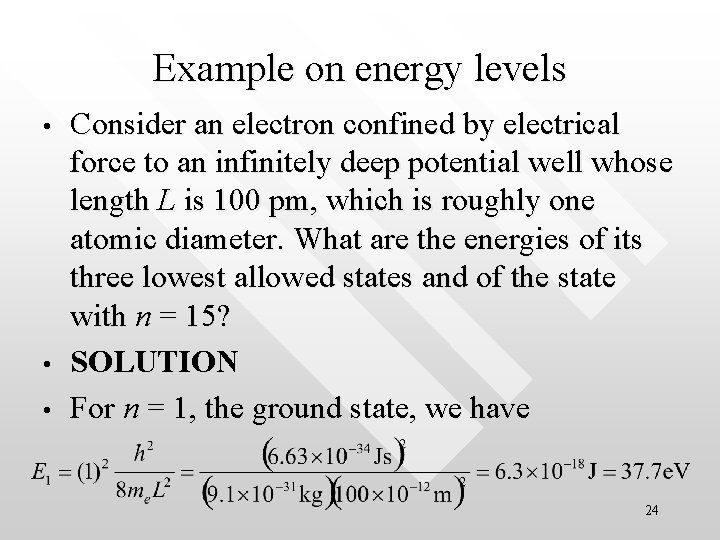Example on energy levels • • • Consider an electron confined by electrical force to an infinitely deep potential well whose length L is 100 pm, which is roughly one atomic diameter. What are the energies of its three lowest allowed states and of the state with n = 15? SOLUTION For n = 1, the ground state, we have 24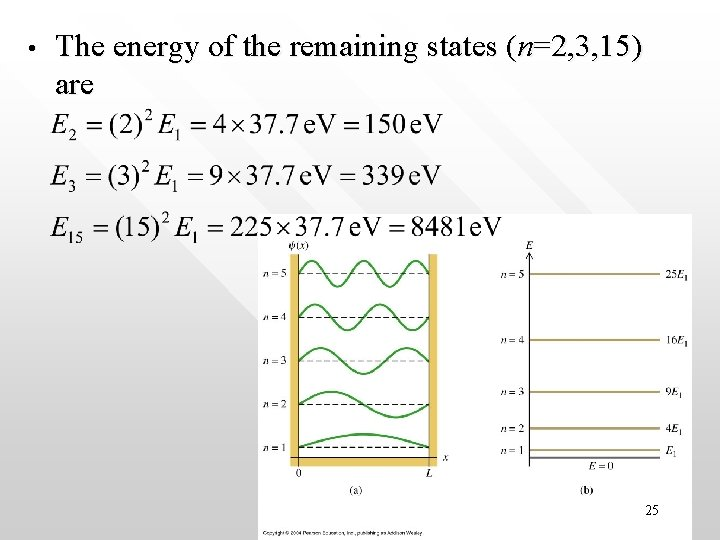• The energy of the remaining states (n=2, 3, 15) are 25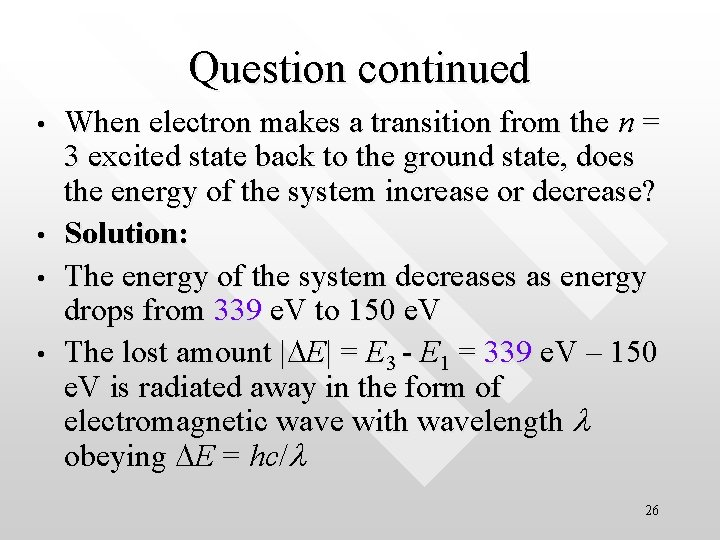Question continued • • When electron makes a transition from the n = 3 excited state back to the ground state, does the energy of the system increase or decrease? Solution: The energy of the system decreases as energy drops from 339 e. V to 150 e. V The lost amount |DE| = E 3 - E 1 = 339 e. V – 150 e. V is radiated away in the form of electromagnetic wave with wavelength l obeying DE = hc/l 26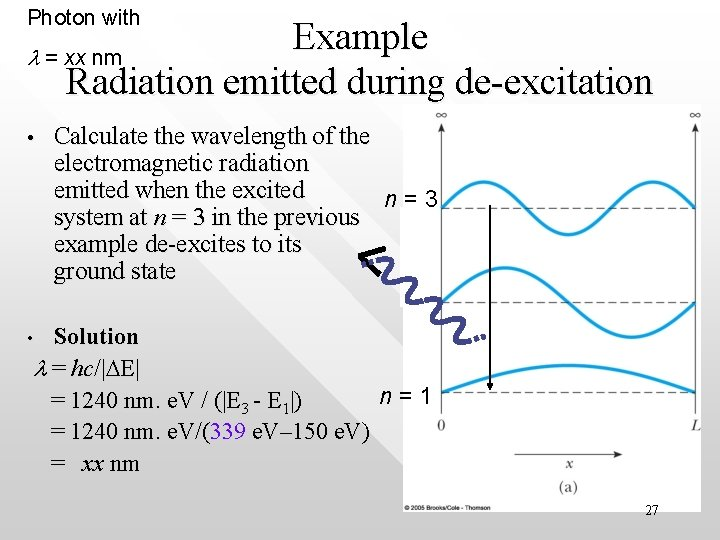Photon with Example l = xx nm Radiation emitted during de-excitation • Calculate the wavelength of the electromagnetic radiation emitted when the excited n=3 system at n = 3 in the previous example de-excites to its ground state Solution l = hc/|DE| n=1 = 1240 nm. e. V / (|E 3 - E 1|) = 1240 nm. e. V/(339 e. V– 150 e. V) = xx nm • 27Example A macroscopic particle’s quantum state • Consider a 1 microgram speck of dust moving back and forth between two rigid walls separated by 0. 1 mm. It moves so slowly that it takes 100 s for the particle to cross this gap. What quantum number describes this motion? 28Solution • The energy of the particle is • Solving for n in • yields • This is a very large number It is experimentally impossible to distinguish between the n = 3 x 1014 and n = 1 + (3 x 1014) states, so that the quantized nature of this motion would never reveal 29 itself •• • The quantum states of a macroscopic particle cannot be experimentally discerned (as seen in previous example) Effectively its quantum states appear as a continuum E(n=1014) = 5 x 10 -22 J DE ≈ 5 x 10 -22/1014 =1. 67 x 10 -36 = 10 -17 e. V is too tiny to the discerned allowed energies in classical system – appear continuous (such as energy carried by a wave; total mechanical energy of an orbiting planet, etc. ) descret energies in quantised system – discrete (such as energy levels in an atom, energies carried by a photon) 30PYQ 4(a) Final Exam 2003/04 • • An electron is contained in a one-dimensional box of width 0. 100 nm. Using the particle-in-abox model, (i) Calculate the n = 1 energy level and n = 4 energy level for the electron in e. V. (ii) Find the wavelength of the photon (in nm) in making transitions that will eventually get it from the n = 4 to n = 1 state Serway solution manual 2, Q 33, pg. 380, modified 31• Solution 4 a(i) In the particle-in-a-box model, standing wave is • The energy of the particle in the box is given by • 4 a(ii) The wavelength of the photon going from n = 4 to n = 1 is l = hc/(E 6 - E 1) = 1240 e. V nm/ (603 – 37. 7) e. V = 2. 2 nm • • formed in the box of dimension L: 32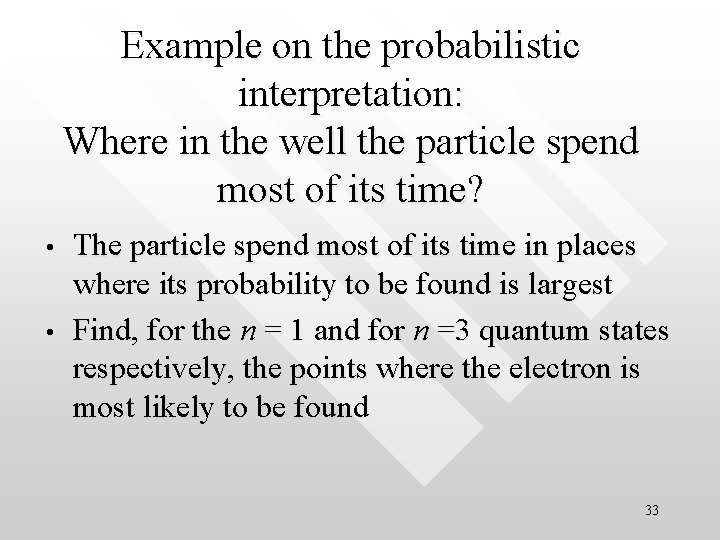Example on the probabilistic interpretation: Where in the well the particle spend most of its time? • • The particle spend most of its time in places where its probability to be found is largest Find, for the n = 1 and for n =3 quantum states respectively, the points where the electron is most likely to be found 33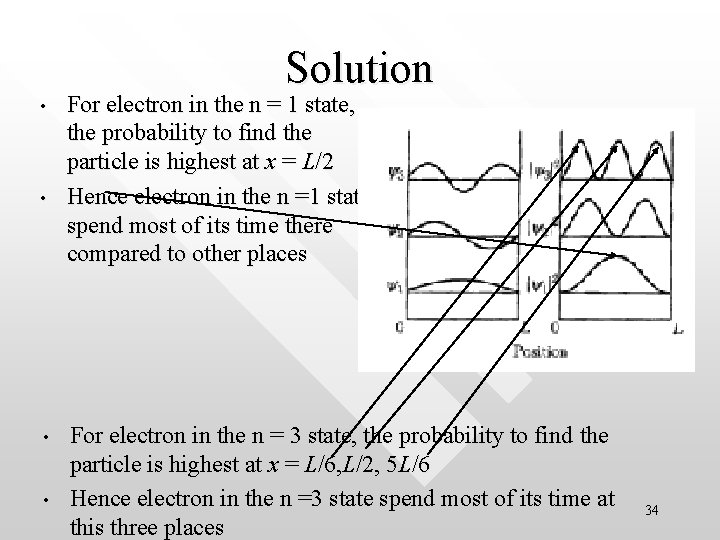Solution • • For electron in the n = 1 state, the probability to find the particle is highest at x = L/2 Hence electron in the n =1 state spend most of its time there compared to other places For electron in the n = 3 state, the probability to find the particle is highest at x = L/6, L/2, 5 L/6 Hence electron in the n =3 state spend most of its time at this three places 34Boundary conditions and normalisation of the wave function in the infinite well • • • Due to the probabilistic interpretation of the wave function, the probability density P(x) = |Y|2 must be such that P(x) = |Y|2 > 0 for 0 < x < L The particle has no where to be found at the boundary as well as outside the well, i. e P(x) = |Y|2 = 0 for x ≤ 0 and x ≥ L 35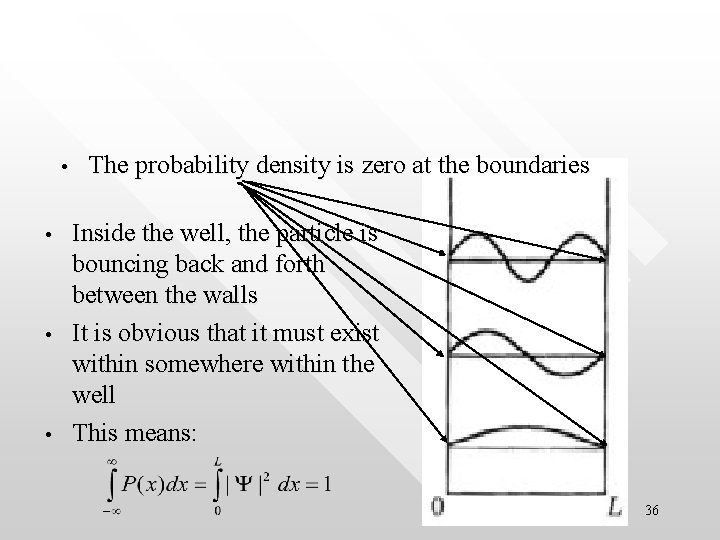• • The probability density is zero at the boundaries Inside the well, the particle is bouncing back and forth between the walls It is obvious that it must exist within somewhere within the well This means: 36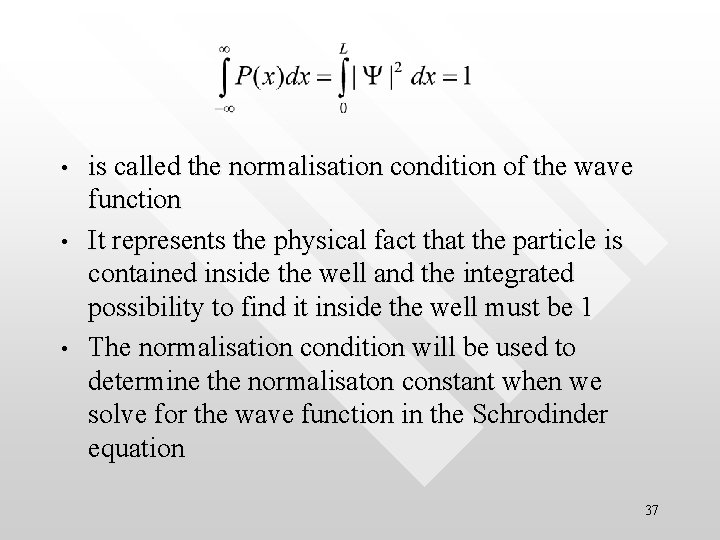• • • is called the normalisation condition of the wave function It represents the physical fact that the particle is contained inside the well and the integrated possibility to find it inside the well must be 1 The normalisation condition will be used to determine the normalisaton constant when we solve for the wave function in the Schrodinder equation 37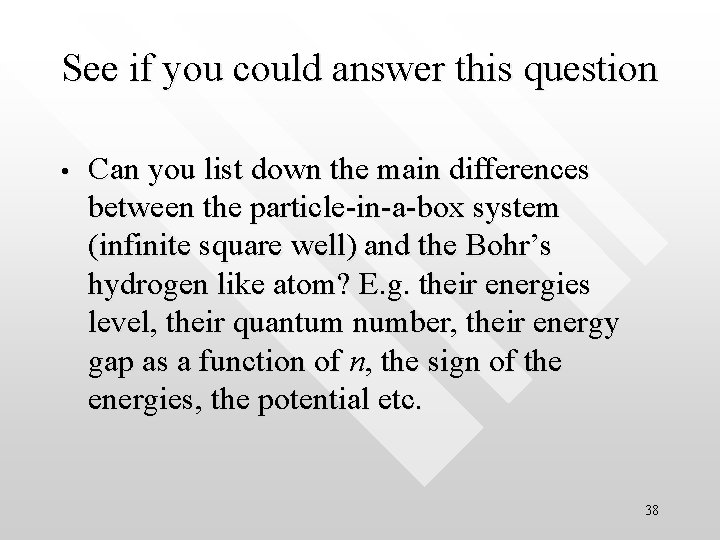See if you could answer this question • Can you list down the main differences between the particle-in-a-box system (infinite square well) and the Bohr’s hydrogen like atom? E. g. their energies level, their quantum number, their energy gap as a function of n, the sign of the energies, the potential etc. 38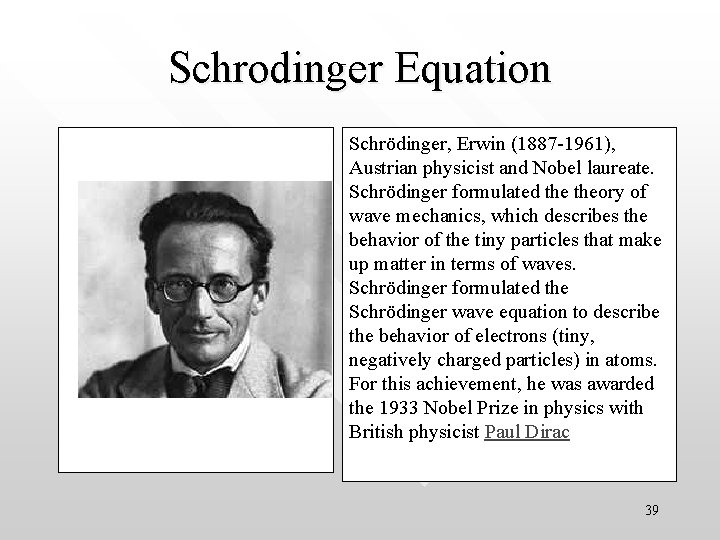Schrodinger Equation Schrödinger, Erwin (1887 -1961), Austrian physicist and Nobel laureate. Schrödinger formulated theory of wave mechanics, which describes the behavior of the tiny particles that make up matter in terms of waves. Schrödinger formulated the Schrödinger wave equation to describe the behavior of electrons (tiny, negatively charged particles) in atoms. For this achievement, he was awarded the 1933 Nobel Prize in physics with British physicist Paul Dirac 39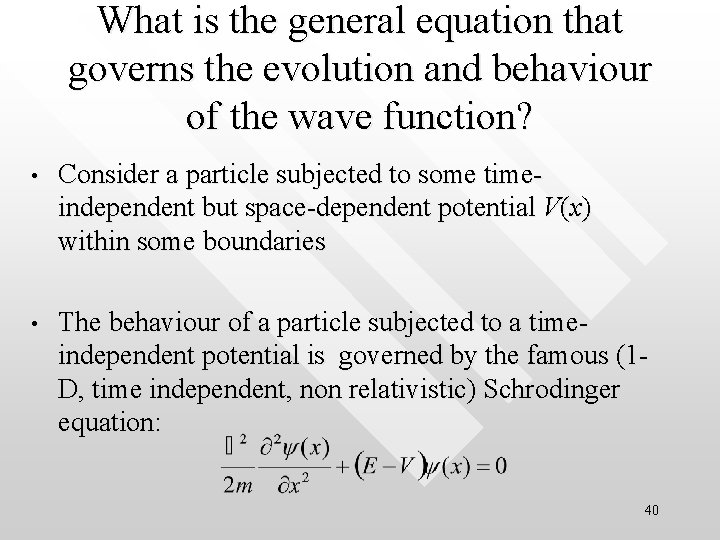What is the general equation that governs the evolution and behaviour of the wave function? • Consider a particle subjected to some timeindependent but space-dependent potential V(x) within some boundaries • The behaviour of a particle subjected to a timeindependent potential is governed by the famous (1 D, time independent, non relativistic) Schrodinger equation: 40How to derive the T. I. S. E • • 1) Energy must be conserved: E = K + U 2) Must be consistent with de Brolie hypothesis that p = h/l 3) Mathematically well-behaved and sensible (e. g. finite, single valued, linear so that superposition prevails, conserved in probability etc. ) Read the msword notes or text books for more technical details (which we will skip here) 41Energy of the particle • The kinetic energy of a particle subjected to potential V(x) is V(x) E, K l • K (= p 2/2 m) = E – V E is conserved if there is no net change in the total mechanical energy between the particle and the surrounding (Recall that this is just the definition of total mechanical energy) • • It is essential to relate the de Broglie wavelength to the energies of the particle: l = h / p = h / √[2 m(E-V)] Note that, as V 0, the above equation reduces to the no-potential case (as we have discussed earlier) 42 l = h / p h / √[2 m. E], where E = K only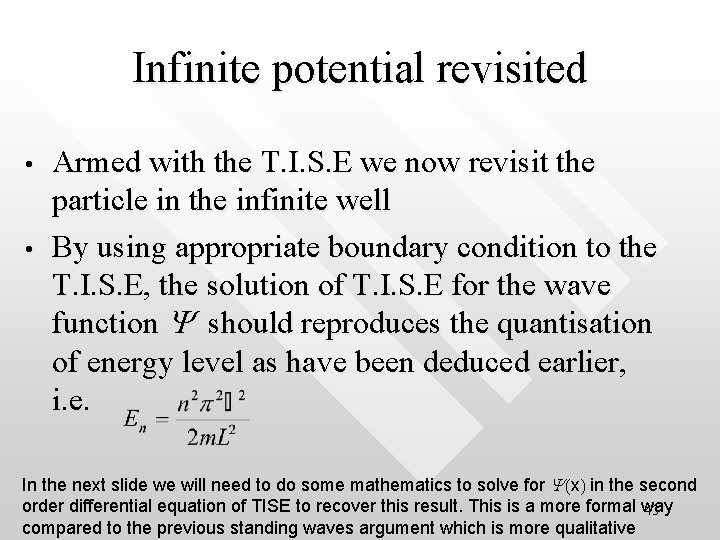Infinite potential revisited • • Armed with the T. I. S. E we now revisit the particle in the infinite well By using appropriate boundary condition to the T. I. S. E, the solution of T. I. S. E for the wave function Y should reproduces the quantisation of energy level as have been deduced earlier, i. e. In the next slide we will need to do some mathematics to solve for Y(x) in the second order differential equation of TISE to recover this result. This is a more formal way 43 compared to the previous standing waves argument which is more qualitative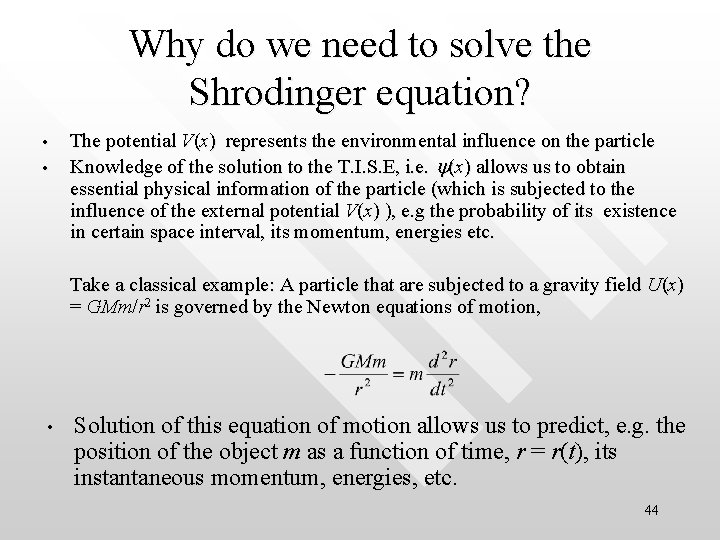Why do we need to solve the Shrodinger equation? • • The potential V(x) represents the environmental influence on the particle Knowledge of the solution to the T. I. S. E, i. e. y(x) allows us to obtain essential physical information of the particle (which is subjected to the influence of the external potential V(x) ), e. g the probability of its existence in certain space interval, its momentum, energies etc. Take a classical example: A particle that are subjected to a gravity field U(x) = GMm/r 2 is governed by the Newton equations of motion, • Solution of this equation of motion allows us to predict, e. g. the position of the object m as a function of time, r = r(t), its instantaneous momentum, energies, etc. 44S. E. is the quantum equivalent of the Newton’s law of motion • • The equivalent of “Newton laws of motion” for quantum particles = Shroedinger equation Solving for the wave function in the S. E. allows us to extract all possible physical information about the particle (energy, expectation values for position, momentum, etc. ) 45The infinite well in the light of TISE Plug the potential function V(x) into the T. I. S. E Within 0 < x < L, V (x) = 0, hence the TISE becomes 46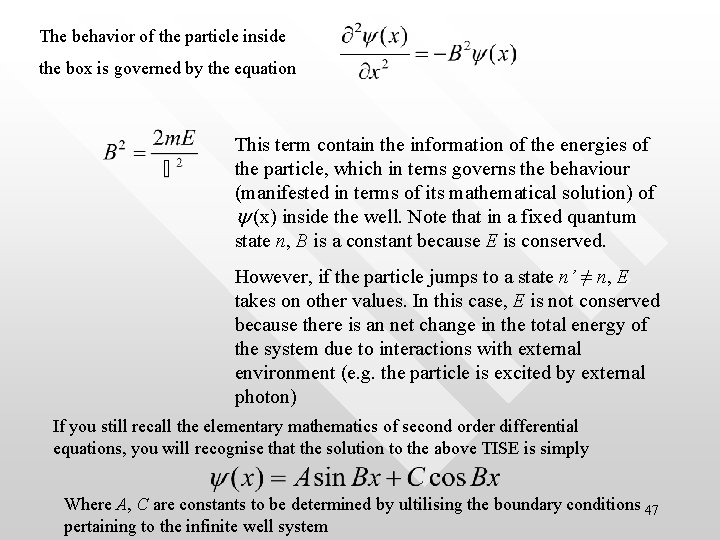The behavior of the particle inside the box is governed by the equation This term contain the information of the energies of the particle, which in terns governs the behaviour (manifested in terms of its mathematical solution) of y (x) inside the well. Note that in a fixed quantum state n, B is a constant because E is conserved. However, if the particle jumps to a state n’ ≠ n, E takes on other values. In this case, E is not conserved because there is an net change in the total energy of the system due to interactions with external environment (e. g. the particle is excited by external photon) If you still recall the elementary mathematics of second order differential equations, you will recognise that the solution to the above TISE is simply Where A, C are constants to be determined by ultilising the boundary conditions 47 pertaining to the infinite well system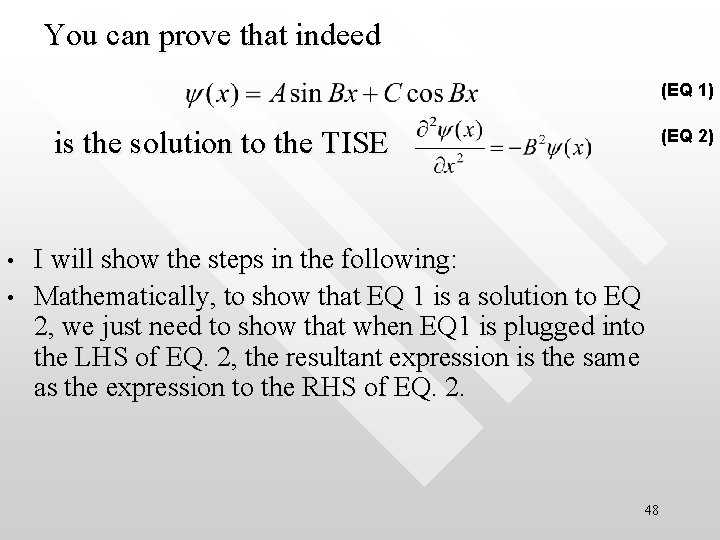You can prove that indeed (EQ 1) is the solution to the TISE • • (EQ 2) I will show the steps in the following: Mathematically, to show that EQ 1 is a solution to EQ 2, we just need to show that when EQ 1 is plugged into the LHS of EQ. 2, the resultant expression is the same as the expression to the RHS of EQ. 2. 48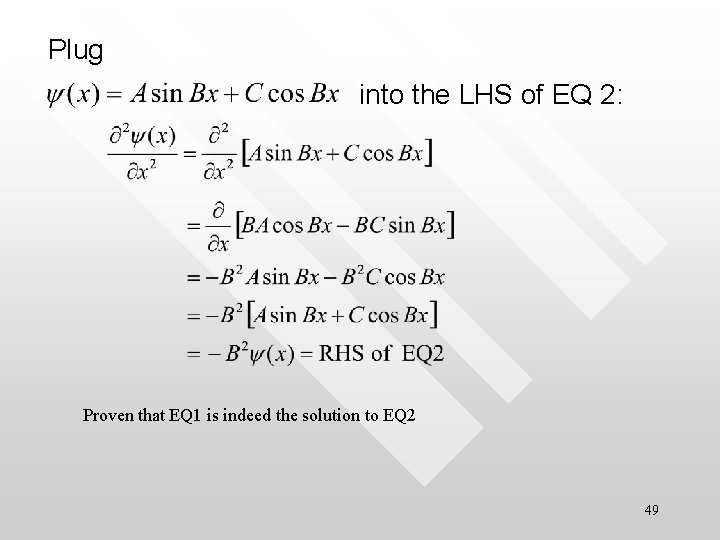Plug into the LHS of EQ 2: Proven that EQ 1 is indeed the solution to EQ 2 49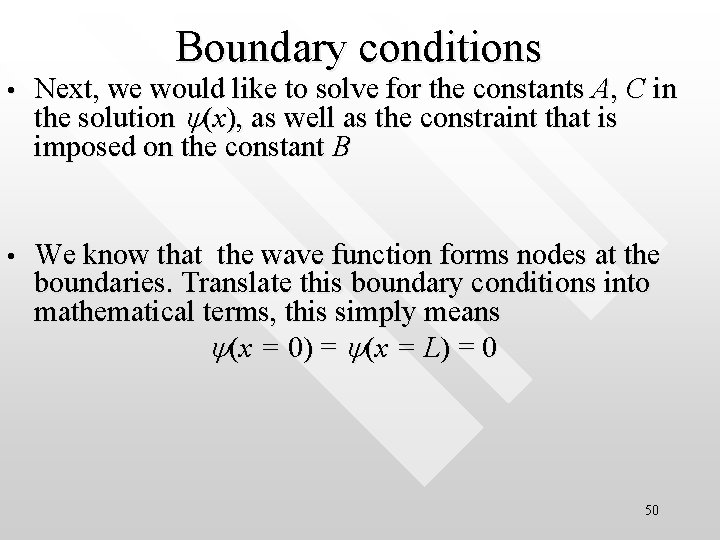Boundary conditions • Next, we would like to solve for the constants A, C in the solution y(x), as well as the constraint that is imposed on the constant B • We know that the wave function forms nodes at the boundaries. Translate this boundary conditions into mathematical terms, this simply means y(x = 0) = y(x = L) = 0 50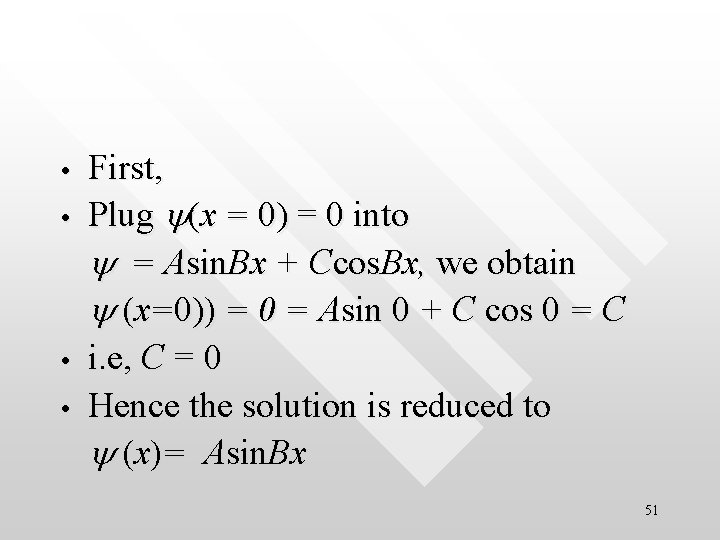• • First, Plug y(x = 0) = 0 into y = Asin. Bx + Ccos. Bx, we obtain y (x=0)) = 0 = Asin 0 + C cos 0 = C i. e, C = 0 Hence the solution is reduced to y (x)= Asin. Bx 51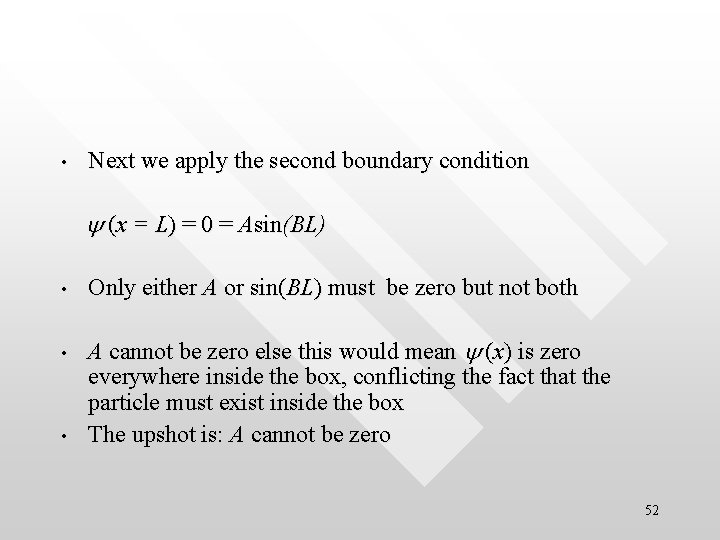• Next we apply the second boundary condition y (x = L) = 0 = Asin(BL) • Only either A or sin(BL) must be zero but not both • A cannot be zero else this would mean y (x) is zero everywhere inside the box, conflicting the fact that the particle must exist inside the box The upshot is: A cannot be zero • 52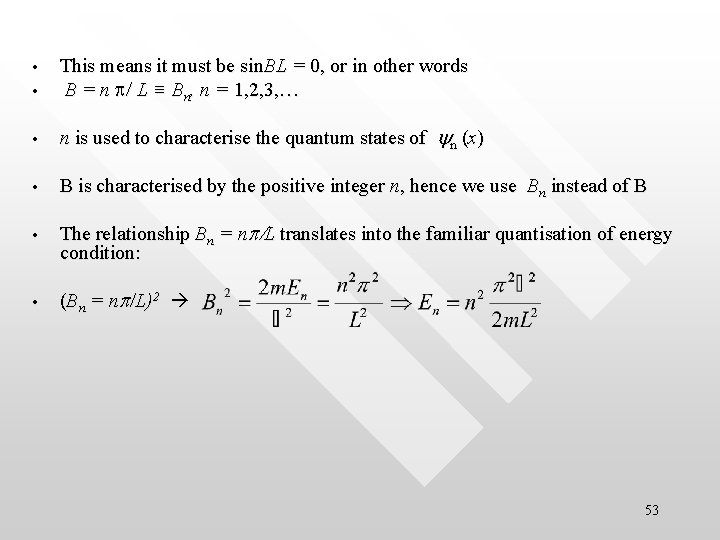• This means it must be sin. BL = 0, or in other words B = n p/ L ≡ Bn, n = 1, 2, 3, … • n is used to characterise the quantum states of yn (x) • B is characterised by the positive integer n, hence we use Bn instead of B • The relationship Bn = np/L translates into the familiar quantisation of energy condition: • (Bn = np/L)2 • 53Ø Hence, up to this stage, the solution is Øyn(x) = Ansin(npx/L), n = 1, 2, 3, …for 0 < x < L Øyn(x) = 0 elsewhere (outside the box) ØThe constant An is yet unknown up to now ØWe can solve for An by applying another The area under the curves of |Yn|2 =1 for every n “boundary condition” – the normalisation condition that: 54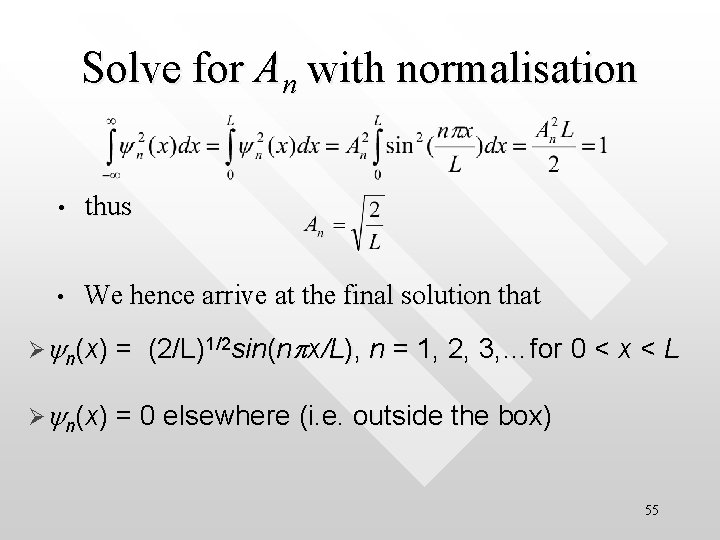Solve for An with normalisation • thus • We hence arrive at the final solution that Øyn(x) = (2/L)1/2 sin(npx/L), n = 1, 2, 3, …for 0 < x < L Øyn(x) = 0 elsewhere (i. e. outside the box) 55• • Example An electron is trapped in a onedimensional region of length L = 1. 0× 10 -10 m. (a) How much energy must be supplied to excite the electron from the ground state to the first state? (b) In the ground state, what is the probability of finding the electron in the region from x = 0. 090 × 10 -10 m to 0. 110 × 1010 m? (c) In the first excited state, what is the probability of finding the electron between x = 0 and x = 0. 250 × 10 -10 m? 0. 25 A 0. 5 A 1 A 56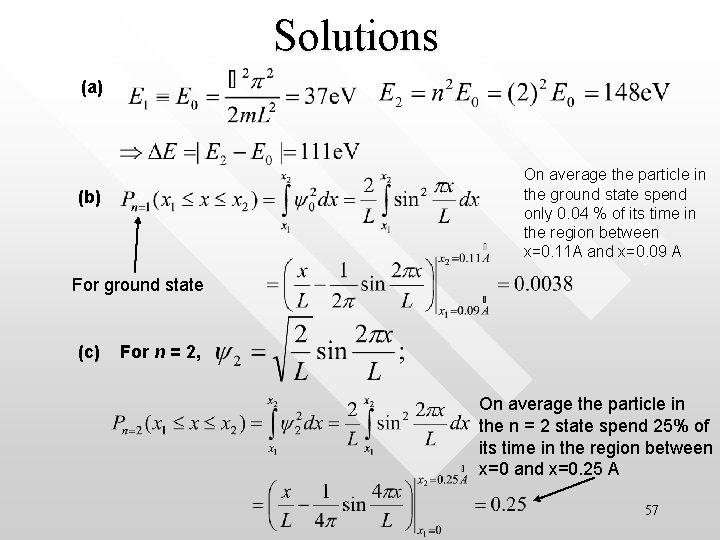Solutions (a) On average the particle in the ground state spend only 0. 04 % of its time in the region between x=0. 11 A and x=0. 09 A (b) For ground state (c) For n = 2, On average the particle in the n = 2 state spend 25% of its time in the region between x=0 and x=0. 25 A 57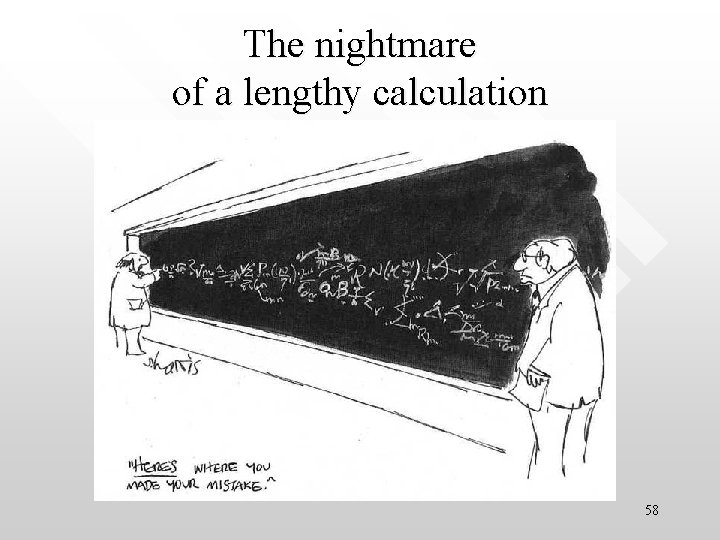The nightmare of a lengthy calculation 58Quantum tunneling • In the infinite quantum well, there are regions where the particle is “forbidden” to V infinity appear I Forbidden region where particle cannot be found because y = 0 everywhere before x < 0 n=1 II III Allowed region where particle can be found Forbidden region where particle cannot be found because y = 0 everywhere after x>L y(x=0)=0 y(x=L)=0 59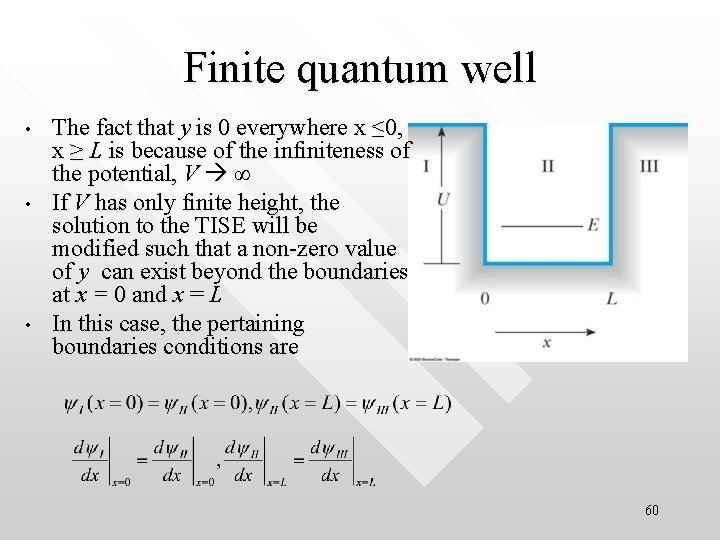Finite quantum well • • • The fact that y is 0 everywhere x ≤ 0, x ≥ L is because of the infiniteness of the potential, V ∞ If V has only finite height, the solution to the TISE will be modified such that a non-zero value of y can exist beyond the boundaries at x = 0 and x = L In this case, the pertaining boundaries conditions are 60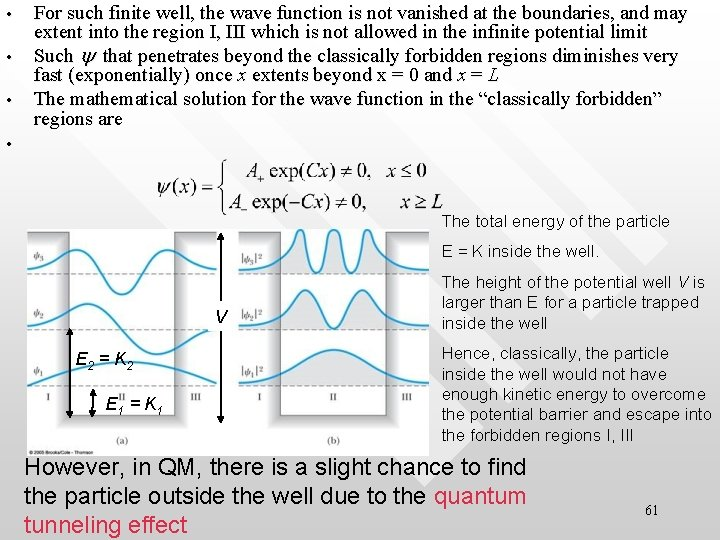• • • For such finite well, the wave function is not vanished at the boundaries, and may extent into the region I, III which is not allowed in the infinite potential limit Such y that penetrates beyond the classically forbidden regions diminishes very fast (exponentially) once x extents beyond x = 0 and x = L The mathematical solution for the wave function in the “classically forbidden” regions are • The total energy of the particle E = K inside the well. V E 2 = K 2 E 1 = K 1 The height of the potential well V is larger than E for a particle trapped inside the well Hence, classically, the particle inside the well would not have enough kinetic energy to overcome the potential barrier and escape into the forbidden regions I, III However, in QM, there is a slight chance to find the particle outside the well due to the quantum tunneling effect 61• E The quantum tunnelling effect allows a confined particle within a finite potential well to penetrate through the classically impenetrable potential wall Hard and high wall, V After many times of banging the wall E Quantum tunneling effect Hard and high wall, V 62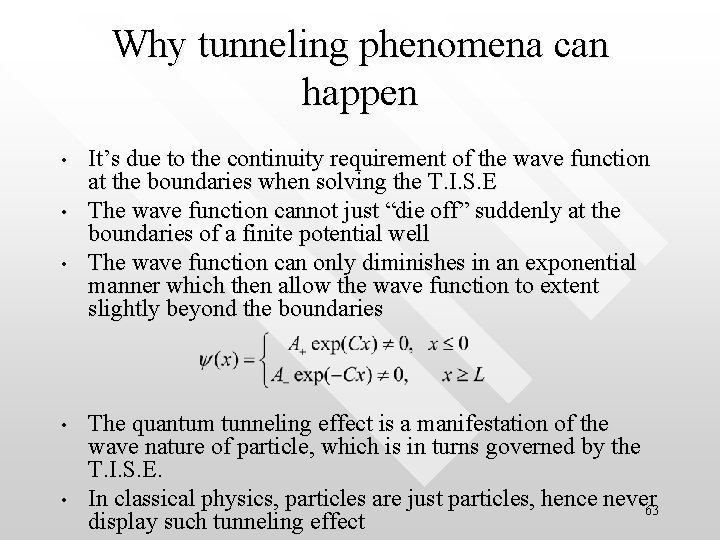Why tunneling phenomena can happen • • • It’s due to the continuity requirement of the wave function at the boundaries when solving the T. I. S. E The wave function cannot just “die off” suddenly at the boundaries of a finite potential well The wave function can only diminishes in an exponential manner which then allow the wave function to extent slightly beyond the boundaries The quantum tunneling effect is a manifestation of the wave nature of particle, which is in turns governed by the T. I. S. E. In classical physics, particles are just particles, hence never 63 display such tunneling effect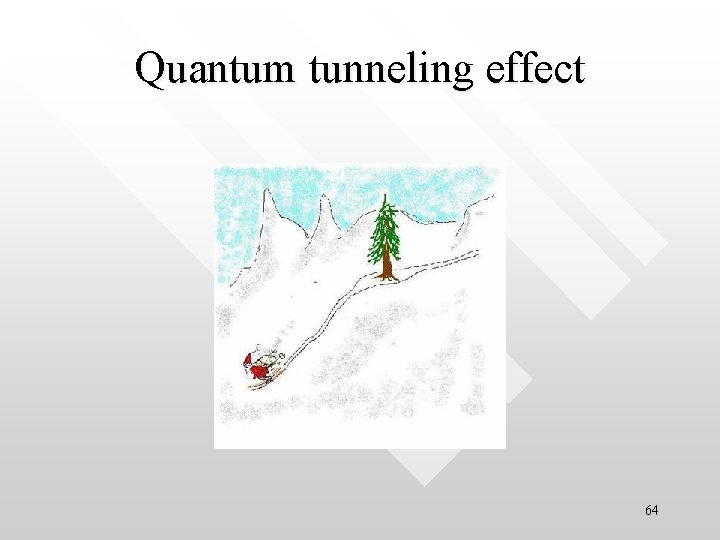Quantum tunneling effect 64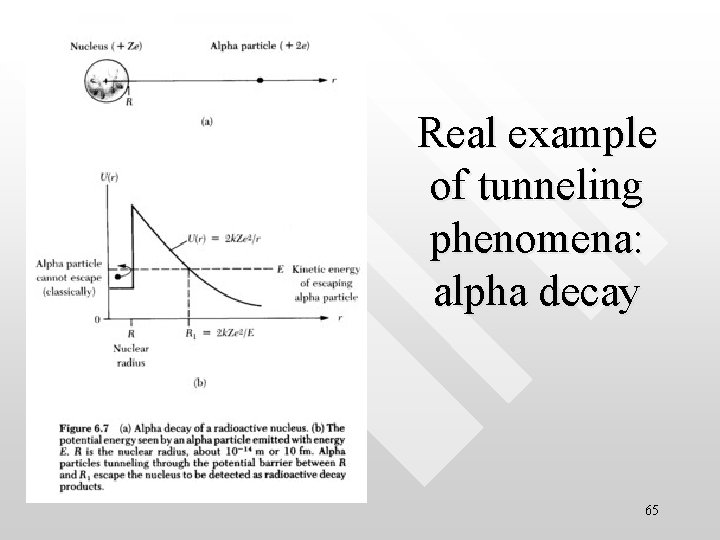Real example of tunneling phenomena: alpha decay 65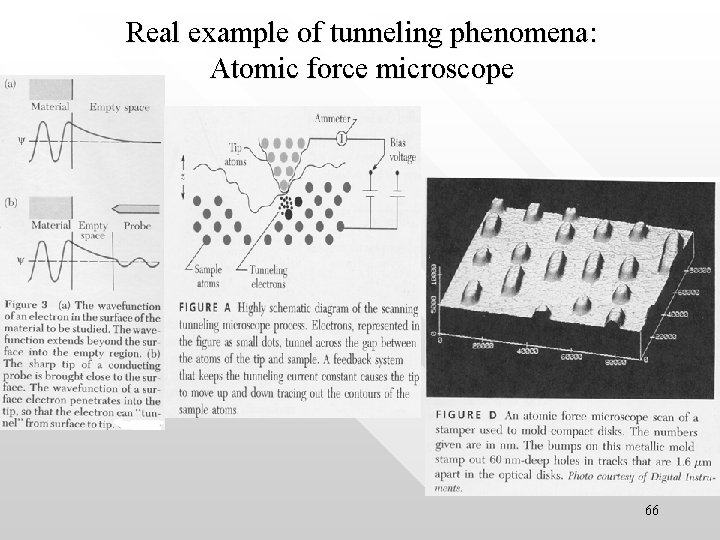Real example of tunneling phenomena: Atomic force microscope 66